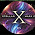## Wednesday, October 11, 2017

### 0-Observation~

EPL- INFORMATION FOR YOUR BUILDING SOUL

### geometry - A movement requires 1 dimension, a rotation requires 2 ...

movement requires 1 dimension, a rotation requires 2 dimensions, a whatrequires three dimensionsMovement A zero-dimensional object cannot move.Aug 1, 2015

Observation
If there is no such thing as zero/0 because nothing which does not produce (entropy/enthalpy), can not exist, then this proves that zero was mock in the first, 'something came from nothing thus goes back into nothing?'. On the other hand if a Sphere is truly zero, and does not move, then the Universe moves around it; IE what is; universe/Universe/UNIVERSE/UNIVERSE/UNIVERSE?

Enthalpy vs Entropy

For the study purposes in chemistry, we divide the universe into two as a system and surrounding. At any time, the part we are interested is the system, and the rest is surrounding. Enthalpy and entropy are two terms describing the reactions taking place in a system and the surrounding. Both enthalpy and entropy are thermodynamic state functions.
What is Enthalpy?
When a reaction takes place, it may absorb or evolve heat, and if the reaction is carried out at constant pressure, this heat is called the enthalpy of the reaction. Enthalpy of molecules cannot be measured. Therefore, change in enthalpy during a reaction is measured. The enthalpy change (∆H) for a reaction in a given temperature and pressure is obtained by subtracting the enthalpy of reactants from the enthalpy of products. If this value is negative, then the reaction is exothermic. If the value is positive, then the reaction is said to be endothermic. The change in enthalpy between any pair of reactants and products is independent of the path between them. Moreover, enthalpy change depends on the phase of the reactants. For example, when the oxygen and hydrogen gases react to produce water vapor, the enthalpy change is -483.7 kJ. However, when the same reactants react to produce liquid water, the enthalpy change is -571.5 kJ.
2H2 (g) +O2 (g) 2H2O (g); ∆H= -483.7 kJ
2H2 (g) +O2 (g) 2H2O (l); ∆H= -571.7 kJ
What is Entropy?
Some things happen spontaneously, others do not. For example, heat will flow from a hot body to a cooler one, but the opposite is never observed even though it does not violate the conservation of energy rule. When a change occurs, the total energy remains constant but is parceled out differently. The direction of change is determined by the distribution of energy. In spontaneous change, things tend to a state in which the energy is more chaotically dispersed. A change is spontaneous if it leads to greater randomness and chaos in the universe as a whole. The degree of chaos, randomness, or dispersal of energy is measured by a state function called the entropy. The second law of thermodynamics is related to entropy, and it says, “the entropy of the universe increases in a spontaneous process.” Entropy is related to the amount of heat generated; that is the extent to which energy has been degraded. In fact, the amount of extra disorder caused by a given amount of heat q depends on the temperature. If it is already very hot, a bit of extra heat doesn’t create much more disorder, but if the temperature is very low, the same amount of heat will cause a dramatic increase in disorder. Therefore, it is more appropriate to write,
ds=dq/T

 What is the difference between Enthalpy and entropy? • Enthalpy is the heat transfer takes place in a constant pressure. Entropy gives an idea of the randomness of a system. • In a reaction, enthalpy change can be positive or negative. Spontaneous reaction takes place in order to increase the universal entropy. • Enthalpy is the energy released or absorbed during a reaction. Enthalpy is the energy surrounded by the system. • Enthalpy is related with the first law of thermodynamics where it says, “Energy can be neither created nor destroyed.” But the entropy is directly related with the second law of thermodynamics.

#### 1 comment:

1.http://chemed.chem.purdue.edu/genchem/topicreview/bp/ch21/gibbs.php

PLEASE COMMENT, OR ADD INFORMATION YOU FEEL PERTAINS